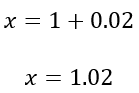Search
• nokwareknight

# Exponential Growth: SAT Practice Test 2, Section 4, #37

Updated: Mar 9This question is looking at exponential growth. Some hints as to how we know this is an exponential growth question include the following:

• A mention of a percent increase or decrease every period

• “Compounded” every certain amount of time

• Variable (x, y, z, t, etc.) in the exponent

But the big giveaway is that the format of the given expression neatly matches the exponential growth formula.Where

• A represents your starting value,

• r represents your growth per period

• t represents the number of periods

Compare this to the following equation:You can see that 1+r in the compound growth formula and x in the expression provided are in the same relative positions.So they are equivalent.We are also told the interest (or growth rate) is 2%. So we can substitute the 2% for r.-Nokware Knight